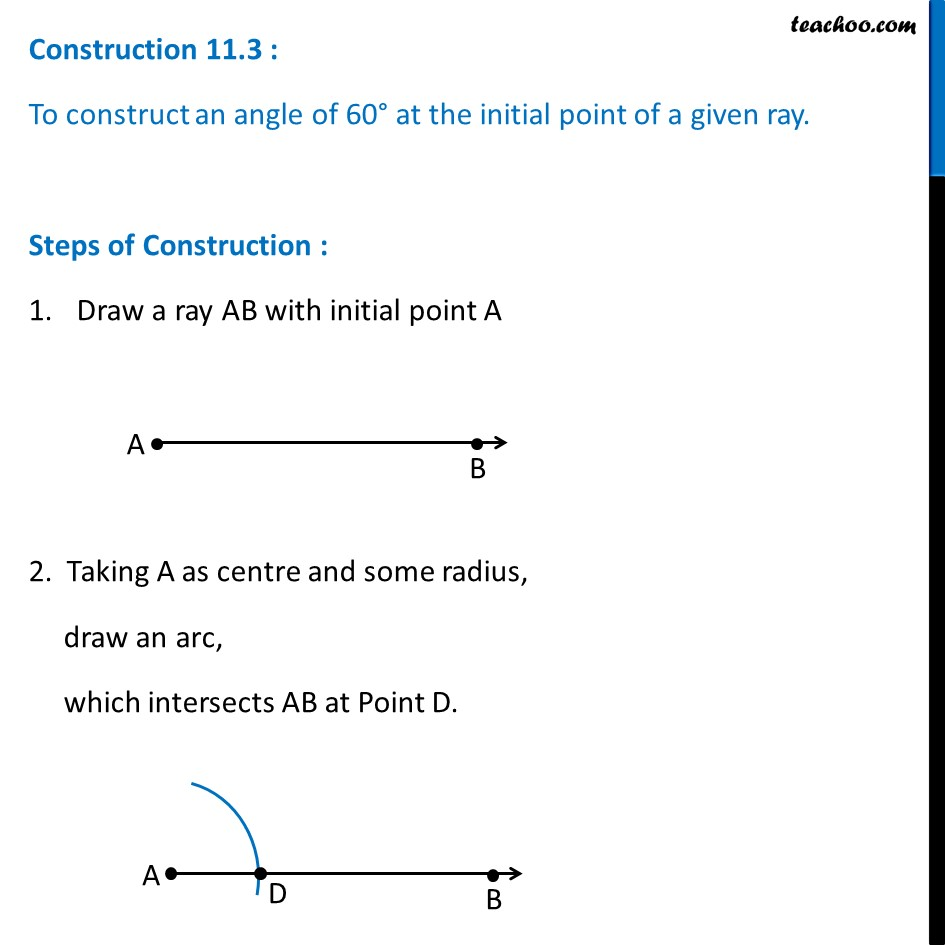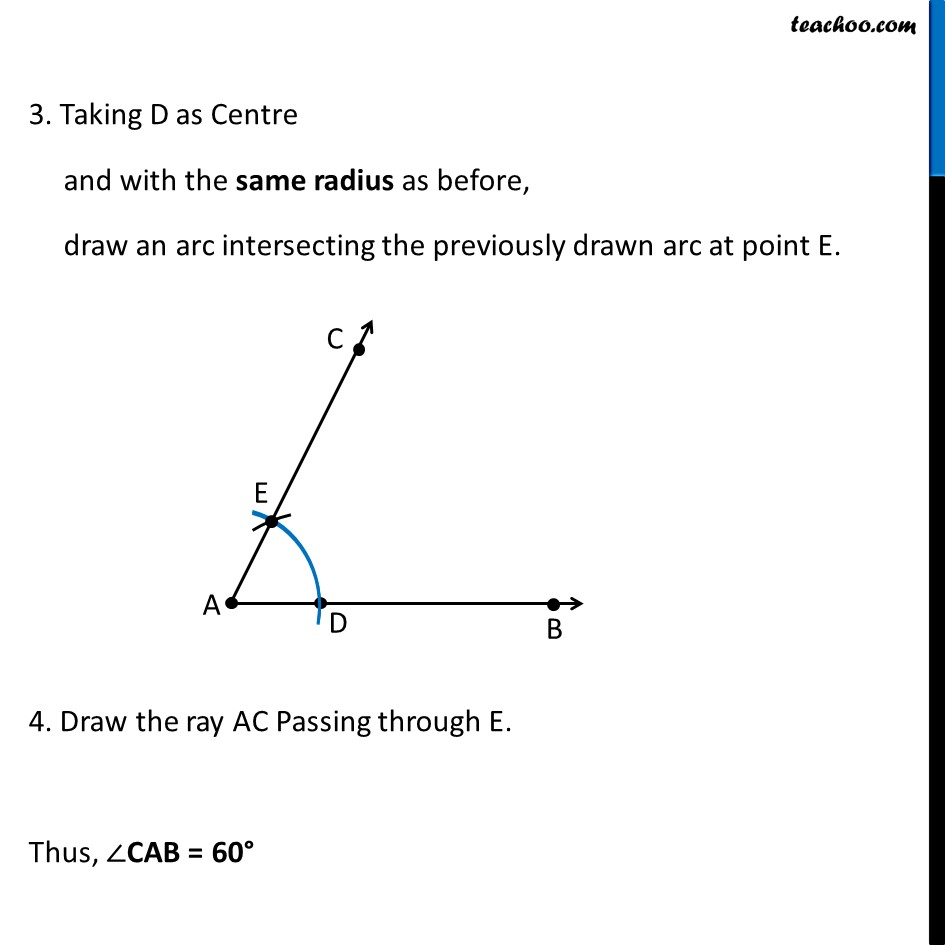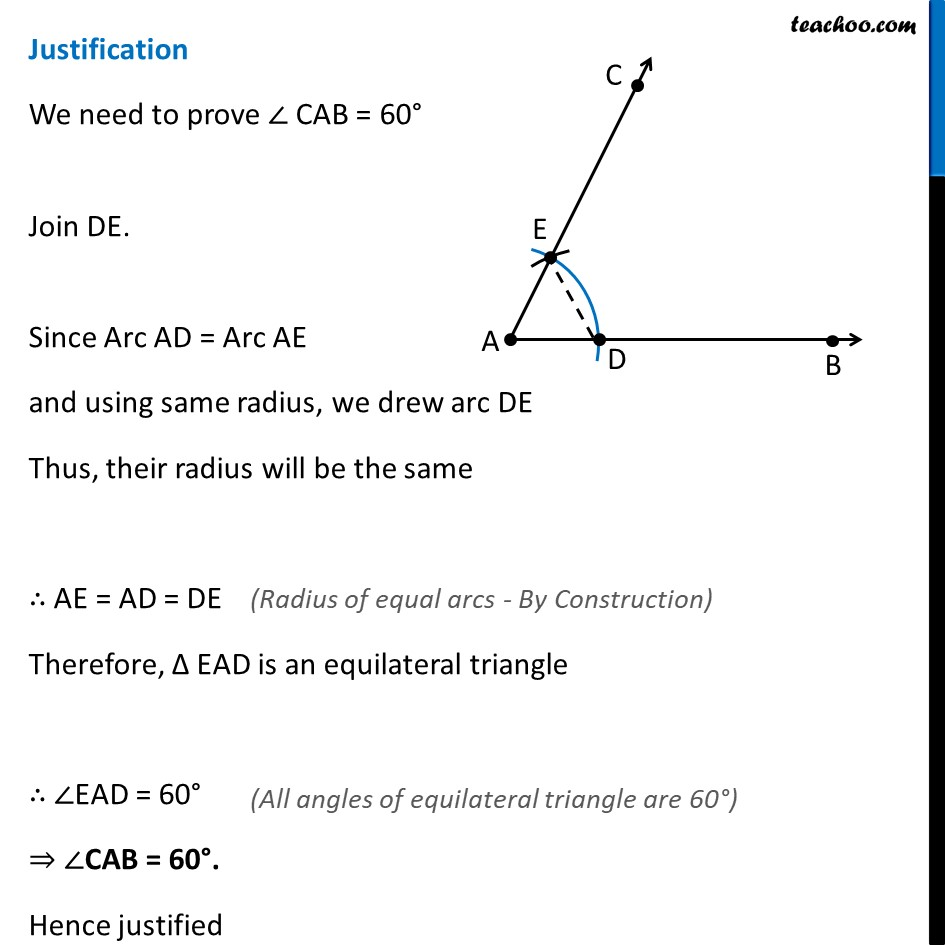Constructing angles - 60, 90, 45, 30 and others

Constructions
Concept wiseLearn in your speed, with individual attention - Teachoo Maths 1-on-1 Class

### Transcript

Construction 11.3 : To construct an angle of 60° at the initial point of a given ray. Steps of Construction : Draw a ray AB with initial point A 2. Taking A as centre and some radius, draw an arc, which intersects AB at Point D. Construction 11.3 : To construct an angle of 60° at the initial point of a given ray. Steps of Construction : Draw a ray AB with initial point A 2. Taking A as centre and some radius, draw an arc, which intersects AB at Point D. 3. Taking D as Centre and with the same radius as before, draw an arc intersecting the previously drawn arc at point E. 4. Draw the ray AC Passing through E. Thus, ∠CAB = 60° Justification We need to prove ∠ CAB = 60° Join DE. Since Arc AD = Arc AE and using same radius, we drew arc DE Thus, their radius will be the same ∴ AE = AD = DE Therefore, ∆ EAD is an equilateral triangle ∴ ∠EAD = 60° ⇒ ∠CAB = 60°. Hence justified (Radius of equal arcs - By Construction) (All angles of equilateral triangle are 60°)